# Code Generation for Prediction of Machine Learning Model Using MATLAB Coder App

This example shows how to generate C/C++ code for the prediction of classification and regression model objects by using the MATLAB® Coder™ app. You can also generate code at the command line using `codegen` (MATLAB Coder). See Code Generation for Prediction of Machine Learning Model at Command Line for details.

Certain classification and regression model objects have a `predict` or `random` function that supports code generation. Prediction using these object functions requires a trained classification or regression model object, but an entry-point function for code generation cannot have these objects as input variables. Work around this limitation by using `saveLearnerForCoder` and `loadLearnerForCoder` as described in this example.

This flow chart shows the code generation workflow for the object functions of classification and regression model objects.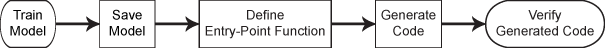In this example, you train a classification ensemble model using k-nearest-neighbor weak learners and save the trained model by using `saveLearnerForCoder`. Then, define an entry-point function that loads the saved model by using `loadLearnerForCoder` and calls the object function. Write a script to test the entry-point function. Finally, generate code by using the MATLAB Coder app and verify the generated code.

### Train Classification Model

Load the `ionosphere` data set. This data set has 34 predictors and 351 binary responses for radar returns, either bad (`'b'`) or good (`'g'`).

`load ionosphere`

Train a classification ensemble model with k-nearest-neighbor weak learners by using the random subspace method. For details of classifications that use a random subspace ensemble, see Random Subspace Classification.

```rng('default') % For reproducibility learner = templateKNN('NumNeighbors',2); Mdl = fitcensemble(X,Y,'Method','Subspace','NPredToSample',5, ... 'Learners',learner,'NumLearningCycles',13);```

### Save Model Using `saveLearnerForCoder`

Save the trained ensemble model to a file named `knnEnsemble.mat` in your current folder.

`saveLearnerForCoder(Mdl,'knnEnsemble')`

`saveLearnerForCoder` makes the full classification model `Mdl` compact, and then saves it to the MATLAB binary file `knnEnsemble.mat` as a structure array in the current folder.

### Define Entry-Point Function

An entry-point function, also known as the top-level or primary function, is a function you define for code generation. You must define an entry-point function that calls code-generation-enabled functions and generate C/C++ code from the entry-point function. All functions within the entry-point function must support code generation.

In a new file in your current folder, define an entry-point function named `myknnEnsemblePredict` that does the following:

• Accept input data (`X`), the file name of the saved model (`fileName`), and valid name-value pair arguments of the `predict` function (`varargin`).

• Load a trained ensemble model by using `loadLearnerForCoder``.`

• Predict labels and corresponding scores from the loaded model.

You can allow for optional name-value arguments by specifying varargin as an input argument. For details, see Code Generation for Variable Length Argument Lists (MATLAB Coder).

`type myknnEnsemblePredict.m % Display the contents of myknnEnsemblePredict.m file. `
```function [label,score] = myknnEnsemblePredict(X,fileName,varargin) %#codegen CompactMdl = loadLearnerForCoder(fileName); [label,score] = predict(CompactMdl,X,varargin{:}); end ```

Add the `%#codegen` compiler directive (or pragma) to the entry-point function after the function signature to indicate that you intend to generate code for the MATLAB algorithm. Adding this directive instructs the MATLAB Code Analyzer to help you diagnose and fix violations that would result in errors during code generation. See Check Code with the Code Analyzer (MATLAB Coder).

Note: If you click the button located in the upper-right section of this page and open this example in MATLAB, then MATLAB opens the example folder. This folder includes the entry-point function file (`myknnEnsemblePredict.m`) and the test file (`test_myknnEnsemblePredict.m`, described later on).

### Set Up Compiler

To generate C/C++ code, you must have access to a C/C++ compiler that is configured properly. MATLAB Coder locates and uses a supported, installed compiler. You can use `mex` `-setup` to view and change the default compiler. For more details, see Change Default Compiler.

### Create Test File

Write a test script that calls the `myknnEnsemblePredict` function. In the test script, specify the input arguments and name-value pair arguments that you use in the generated code. You use this test script to define input types automatically when generating code using the MATLAB Coder app.

In this example, create the `test_myknnEnsemblePredict.m` file in your current folder, as shown.

`type test_myknnEnsemblePredict.m % Display the contents of test_myknnEnsemblePredict.m file. `
```%% Load Sample data load ionosphere %% Test myknnEnsemblePredict [label,score] = myknnEnsemblePredict(X,'knnEnsemble','Learners',1:13); ```

For details, see Automatically Define Input Types by Using the App (MATLAB Coder).

### Generate Code Using MATLAB Coder App

The MATLAB Coder app generates C or C++ code from MATLAB code. The workflow-based user interface steps you through the code generation process. The following steps describe a brief workflow of the MATLAB Coder App. For more details, see MATLAB Coder (MATLAB Coder) and Generate C Code by Using the MATLAB Coder App (MATLAB Coder).

1. Open the MATLAB Coder App and Select the Entry-Point Function File.

On the Apps tab, in the Apps section, click the Show more arrow to open the apps gallery. Under Code Generation, click MATLAB Coder. The app opens the Select Source Files page. Enter or select the name of the entry-point function, `myknnEnsemblePredict`.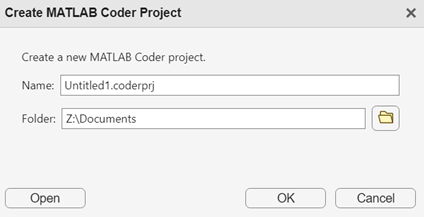Click Next to go to the Define Input Types page.

2. Define Input Types

Because C uses static typing, MATLAB Coder must determine the properties of all variables in the MATLAB files at compile time. Therefore, you need to specify the properties of the entry-point function inputs.

Enter or select the test script `test_myknnEnsemblePredict` and click Autodefine Input Types.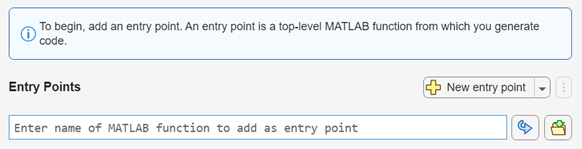The MATLAB Coder app recognizes input types of the `myknnEnsemblePredict` function based on the test script.

Modify the input types:

• `X` — The app infers that input `X` is `double(351x34)`. The number of predictors must be fixed to be the same as the number of predictors in the trained model. However, you can have a different number of observations for prediction. If the number of observations is unknown, change `double(351x34)` to `double(:351x34)` or `double(:infx34)`. The setting `double(:351x34)` allows the number of observations up to 351, and the setting `double(:infx34)` allows an unbounded number of observations. In this example, specify `double(:infx34)` by clicking `351` and selecting `:inf`.

• `fileName` — Click `char`, select Define Constant, and type the file name with single quotes, `'knnEnsemble'`.

• `varargin{1}` — Names in name-value pair arguments must be compile-time constants. Click `char`, select Define Constant, and type `'Learners'`.

• `varargin{2}` — To allow user-defined indices up to 13 weak learners in the generated code, change `double(1x13)` to `double(1x:13)`.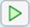Click Next to go to the Check for Run-Time Issues page. This optional step generates a MEX file, runs the MEX function, and reports issues. Click Next to go to the Generate Code page.

3. Generate C Code

Set Build type to MEX and click Generate. The app generates a MEX function, `myknnEnsemblePredict_mex`. A MEX function is a C/C++ program that is executable from MATLAB. You can use a MEX function to accelerate MATLAB algorithms and to test the generated code for functionality and run-time issues. For details, see MATLAB Algorithm Acceleration (MATLAB Coder) and Why Test MEX Functions in MATLAB? (MATLAB Coder).

Depending on the specified build type, MATLAB Coder generates a MEX function or standalone C/C++ code compiled to a static library, dynamic linked library, or executable. For details on setting a build type, see Configure Build Settings (MATLAB Coder).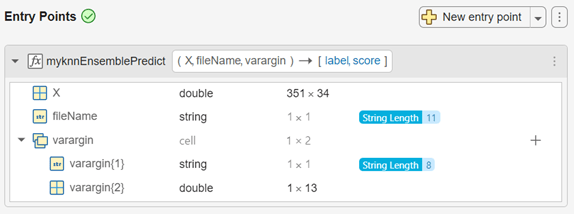Click Next to go to the Finish Workflow page.

4. Review the Finish Workflow Page

The Finish Workflow page indicates that code generation succeeded. This page also provides a project summary and links to generated output.### Generate Code Using Script

You can convert a MATLAB Coder project to the equivalent script of MATLAB commands after you define input types. Then you run the script to generate code. For details, see Convert MATLAB Coder Project to MATLAB Script (MATLAB Coder).

On the MATLAB Coder app toolbar, click the Open action menu button: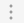Select Convert to script, and then click Save. The app creates the file `myknnEnsemblePredict_script.m`, which reproduces the project in a configuration object and runs the `codegen` (MATLAB Coder) function.

Display the contents of the file `myknnEnsemblePredict_script.m`.

`type myknnEnsemblePredict_script.m`
```% MYKNNENSEMBLEPREDICT_SCRIPT Generate MEX-function myknnEnsemblePredict_mex % from myknnEnsemblePredict. % % Script generated from project 'myknnEnsemblePredict.prj' on 17-Nov-2017. % % See also CODER, CODER.CONFIG, CODER.TYPEOF, CODEGEN. %% Create configuration object of class 'coder.MexCodeConfig'. cfg = coder.config('mex'); cfg.GenerateReport = true; cfg.ReportPotentialDifferences = false; %% Define argument types for entry-point 'myknnEnsemblePredict'. ARGS = cell(1,1); ARGS{1} = cell(4,1); ARGS{1}{1} = coder.typeof(0,[Inf 34],[1 0]); ARGS{1}{2} = coder.Constant('knnEnsemble'); ARGS{1}{3} = coder.Constant('Learners'); ARGS{1}{4} = coder.typeof(0,[1 13],[0 1]); %% Invoke MATLAB Coder. codegen -config cfg myknnEnsemblePredict -args ARGS{1} -nargout 2 ```

Run the script.

`myknnEnsemblePredict_script`
```Code generation successful: To view the report, open('codegen/mex/myknnEnsemblePredict/html/report.mldatx') ```

### Verify Generated Code

Test a MEX function to verify that the generated code provides the same functionality as the original MATLAB code. To perform this test, run the MEX function using the same inputs that you used to run the original MATLAB code, and then compare the results. Running the MEX function in MATLAB before generating standalone code also enables you to detect and fix run-time errors that are much harder to diagnose in the generated standalone code. For more details, see Why Test MEX Functions in MATLAB? (MATLAB Coder).

Pass some predictor data to verify that `myknnEnsemblePredict` and the MEX function return the same results.

```[label1,score1] = predict(Mdl,X,'Learners',1:10); [label2,score2] = myknnEnsemblePredict(X,'knnEnsemble','Learners',1:10); [label3,score3] = myknnEnsemblePredict_mex(X,'knnEnsemble','Learners',1:10);```

Compare `label1`, `label2`, and `label3` by using `isequal`.

`isequal(label1,label2,label3)`
```ans = logical 1 ```

`isequal` returns logical 1 (`true`), which means all the inputs are equal.

The `score3` output from the MEX function might include round-off differences compared with the output from the `predict` function. In this case, compare `score1` and `score3`, allowing a small tolerance.

`find(abs(score1-score3) > 1e-12)`
```ans = 0x1 empty double column vector ```

`find` returns an empty vector if the element-wise absolute difference between `score1` and `score3` is not larger than the specified tolerance `1e-12`. The comparisons confirm that `myknnEnsemblePredict` and the MEX function return the same results.